### Home > PC > Chapter 4 > Lesson 4.1.1 > Problem4-13

4-13.
1. Convert the following angle measures. Use exact values. Homework Help ✎

3.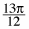radians to degrees

4.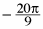radians to degrees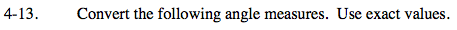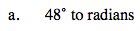$\frac{\text{degrees}}{180\degree}=\frac{\text{radians}}{\pi}$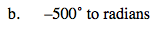$\frac{500\degree}{180\degree}=\frac{\text{radians}}{\pi}$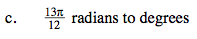$\frac{\text{degrees}}{180\degree}=\frac{\frac{13\pi}{12}}{\pi}$

$\frac{\text{degrees}}{180\degree}=\frac{13}{12}$

$\text{degrees }=\frac{(13)(180\degree)}{12}$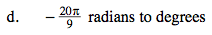$\frac{\text{degrees}}{180\degree}=\frac{\text{radians}}{\pi}$